## How to Calculate and Solve for Velocity | Motion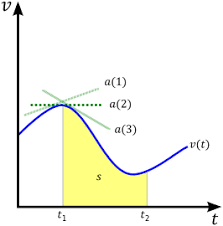The image above represents velocity.

To compute for velocity, three essential parameters are needed and these parameters are angular velocity (ω), amplitude (A) and displacement (y).

The formula for calculating velocity:

v = ω√(A² – y²)

Where;

v = Velocity
ω = Angular Velocity
A = Amplitude
y = Displacement

Let’s solve an example;
Find the velocity when the angular velocity is 7, amplitude is 10 and displacement is 13.

This implies that;

ω = Angular Velocity = 7
A = Amplitude = 10
y = Displacement = 13

v = ω√(A² – y²)
v = 7 x √(10² – 13²)
v = 7 x √(100 – 169)
v = 7 x √(-69)

i signifies a complex number = √(-1).

v = 7 x √(69) x √(-1)
v = 7 x √(69)i
v = 7 x 8.30i
v = 7(8.30i)

Therefore, the velocity is 7(8.30i) m/s.

Calculating the Angular Velocity when the Velocity, the Amplitude and the Displacement is Given.

ω = v / √(A2 – y2)

Where;

ω = Angular Velocity
v = Velocity
A = Amplitude
y = Displacement

Let’s solve an example;
Find the angular velocity when the velocity is 28, the amplitude is 14 and the displacement is 10.

This implies that;

v = Velocity = 28
A = Amplitude = 14
y = Displacement = 10

ω = v / √(A2 – y2)
ω = 28 / √(142 – 102)
ω = 28 / √(196 – 100)
ω = 28 / √(96)
ω = 28 / 9.79
ω = 2.86

Therefore, the angular velocity is 2.86.

## How to Calculate and Solve for Angular Velocity | Motion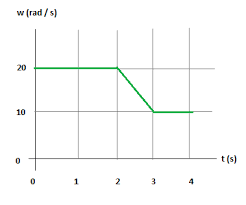The image above represents angular velocity.

To compute for angular velocity, two essential parameters are needed and these parameters are mass (m) and elastic constant (k).

The formula for calculating angular velocity:

ω = √(k / m)

Where;

ω = Angular Velocity
k = Elastic Constant
m = Mass

Let’s solve an example;
Find the angular velocity when the mass is 11 and the elastic constant is 28.

This implies that;

k = Elastic Constant = 28
jm = Mass = 11

ω = √(k / m)
ω = √(28 / 11)
ω = √(2.54)
ω = 1.59

Therefore, the angular velocity is 1.59 rad/s.

Calculating the Elastic Constant when the Angular Velocity and the Mass is Given.

k = ω2m

Where;

k = Elastic Constant
ω = Angular Velocity
m = Mass

Let’s solve an example;
Find the elastic constant when the angular velocity is 12 and the mass is 8.

This implies that;

ω = Angular Velocity = 12
m = Mass = 8

k = ω2m
k = 1228
k = 144 x 8
k = 1152

Therefore, the elastic constant is 1152.

## How to Calculate and Solve for Angular Velocity | Motion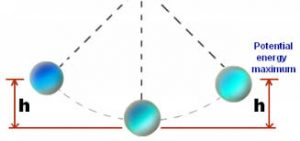The image above represents angular velocity.

To Compute for angular velocity, one essential parameter is needed and the parameter is frequency (f).

The formula for calculating angular velocity:

ω = 2πf

Where;

ω = Angular Velocity
f = Frequency

Let’s solve an example;
Find the angular velocity when the frequency is 18.

This implies that;

f = frequency = 18

ω = 2πf
ω = 2 x π x 18
ω = 113.09

Therefore, the angular velocity is 113.09 rad/s.

## How to Calculate and Solve for Acceleration | Motion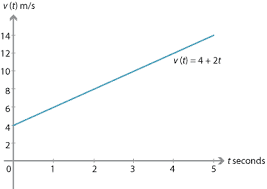The image above represents acceleration.

To compute for acceleration, two essential parameters are needed and these parameters are angular velocity (ω) and radius (r).

The formula for calculating acceleration:

a = ω²r

Where;

a = Acceleration
ω = Angular Velocity

Let’s solve an example;
Given that the angular velocity is 24 and the radius is 12. Find the acceleration?

This implies that;

ω = Angular Velocity = 24

a = ω²r
a = 24² x 12
a = 576 x 12
a = 6912

Therefore, the acceleration is 6912 m/s².

Calculating the Angular Velocity when the Acceleration and the Time is Given.

ω = √a / r

Where;

ω = Angular Velocity
a = Acceleration

Let’s solve an example;
Find the angular velocity when the acceleration is 50 with a radius of 2.

This implies that;

a = Acceleration = 50

ω = √a / r
ω = √50 / 2
ω = √25
ω = 5

Therefore, the angular velocity is 5.

## How to Calculate and Solve for Velocity | Motion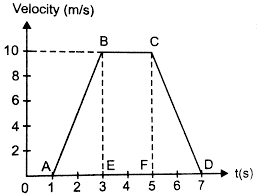The image above represents velocity.

To compute for velocity, two essential parameters are needed and these parameters are angular velocity (ω) and radius (r).

The formula for calculating velocity:

v = ωr

Where;

v = Velocity
ω = Angular Velocity

Let’s solve an example;
Find the velocity when the angular velocity is 17 with a radius of 6.

This implies that;

ω = Angular Velocity = 17

v = ωr
v = 17 x 6
v = 102

Therefore, the velocity is 102 m/s.

Calculating the Angular Velocity when the Velocity and the Radius is Given.

ω =v / r

Where;

ω = Angular Velocity
v = Velocity

Let’s solve an example;
Given that the velocity is 30 with a radius of 3. Find the angular velocity?

This implies that;

v = Velocity = 30

ω = v / r
ω = 30 / 3
ω = 10

Therefore, the angular velocity is 10.

## How to Calculate and Solve for Centripetal Acceleration, Angular Velocity and Radius of Circular Path of a Body | The Calculator Encyclopedia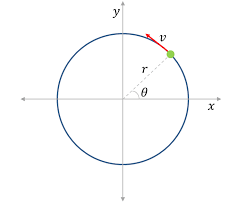The image above represents centripetal acceleration in a motion of circular path.

To compute for the centripetal acceleration, two essential parameters are needed and these parameters are angular velocity (ω) and radius of circular path (r).

The formula for the calculating centripetal acceleration:

a = ω²r

Where:
a = Centripetal Acceleration
ω = Angular Velocity
r = Radius of Circular Path

Let’s solve an example;
Find the centripetal acceleration with an angular velocity of 33 and a radius of 21.

This implies that;
ω = Angular Velocity = 33
r = Radius of Circular Path = 21

a = ω²r
a = 33² x 21
a = 1089 x 21
a = 22869

Therefore, the centripetal acceleration is 22869 m/s².

Calculating the Angular Velocity when Centripetal Acceleration and Radius of Circular path is Given.

ω = √a / r

Where:
ω = Angular Velocity
a = Centripetal Acceleration
r = Radius of Circular Path

Let’s solve an example;
Find the angular velocity with a centripetal acceleration of 320 and a radius of 8.

This implies that;
a = Centripetal Acceleration = 320
r = Radius of Circular Path = 8

ω = √a / r
ω = √320 / 8
ω = √40
ω = 6.32

Therefore, the angular velocity is 6.32.

## How to Calculate and Solve for Number of Revolutions per Minute and Angular Velocity of Motion of Circular Path | The Calculator Encyclopedia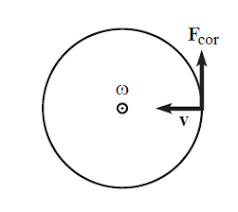The image above represent angular velocity.

To compute the angular velocity, one essential parameter is needed and its parameter is Number of Revolutions per Minute (N).

The formula for calculating angular velocity:

ω = 2πN / 60

Where;
ω = Angular velocity
N = Number of revolutions per minute

Let’s solve an example;
Find the Angular Velocity with a number of revolutions per minute as 60.

This implies that;
N = Number of revolutions per minute = 60

ω = 2πN / 60
ω = 2 x π x 24 / 60
ω = 150.816/ 60
ω = 2.5136

Therefore, the angular velocity is 2.5136 rad/s.

Calculating the Number of Revolutions per Minute when Angular Velocity is Given.

N = ω60 /

Where;
N = Number of revolutions per minute
ω = Angular velocity

Let’s solve an example;
With an angular velocity of 40. Find the number of revolutions per minute?

This implies that;
ω = Angular velocity = 40

N = ω60 /
N = 40 x 60 / 6.284
N = 2400 / 6.284
N = 381.9

Therefore, the number of revolutions per minute is 381.9 min.

## How to Calculate and Solve for Mass, Angular Velocity, Radius and Centrifugal Force of a Body | The Calculator Encyclopedia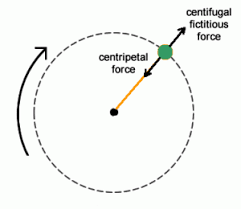The image above represents the centrifugal force.

To compute for the centrifugal force, three essential parameters are needed and these parameters are Mass of the body (m), Angular Velocity of the body (w) and Radius (r).

The formula for calculating the centrifugal force:

F = mω²r

Where:
F = Centrifugal Force
m = mass of the body
ω = angular velocity

Let’s solve an example;
Find the centrifugal force with mass of the body as 12, angular velocity as 32 and a radius of 8.

This implies that;
m = mass of the body = 12
ω = angular velocity = 32

F = mω²r
F = 12 x 32² x 8
F = 12 x 1024 x 8
F = 98304

Therefore, the centrifugal force is 98304 N.

Calculating the Mass of the body (m) when the Centrifugal Force, Angular Velocity and Radius is Given.

m = F / w2r

Where;
m = mass of the body
F = Centrifugal Force
ω = angular velocity

Let’s solve an example;
Find the mass of a body when centrifugal force is 140 with an angular velocity of 24 and a radius of 10.

This implies that;
F = Centrifugal Force = 140
ω = angular velocity = 24